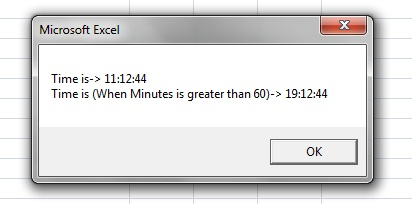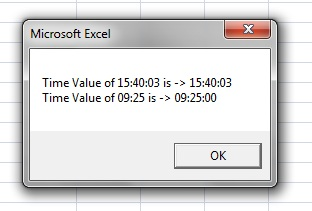# VBA-Excel: Date-Time Functions – TimeSerial() and TimeValue()

TimeSerial()

`Description:`

The TimeSerial() function returns the Time type based on the parameters provided (Hours, Minutes and Seconds).

`Format:`

DateSerial(Hour,Minutes,Seconds)

`Arguments:`
• Hour
• Manda­tory
• Type: Numeric or Any Numeric Expression
• Between 0 and 23
• Minutes
• Manda­tory
• Type: Numeric or Any Numeric Expression
• If it’s greater than 60, than respective number will be added to the Hour, Example say 65, means Hours will be increased by one and Minutes will be 5
• Seconds
• Manda­tory
• Type: Numeric or Any Numeric Expression
• If it’s greater than 60, than respective number will be added to the Minutes, Example say 70, means Minutes will be increased by one and Seconds will be 10

Example:

```Function FnTimeSerial()

Dim varHour

Dim varMinute

Dim varSecond

Dim strResult

<b>varHour = "11"</b>

<b>    varMinute = "12"</b>

<b>      varSecond = "44"</b>

strTime = TimeSerial(varHour, varMinute, varSecond)

strResult = "Time is-&gt; " &amp; strTime &amp; vbCrLf

varHour = "18"

varMinute = "72"

varSecond = "44"

strTime =TimeSerial(varHour, varMinute, varSecond)

strResult = strResult &amp; "Time is (<b>When Minutes is greater than 60</b>)-&gt; " &amp; strTime

MsgBox strResult
```

End FunctionThe TimeSerial() function returns the Time type based on the parameters provided (Hours, Minutes and Seconds).

______________________________________________________________________________

TimeValue()

`Description:`

The DateValue() function converts string to a date which contains time and returns it.

`Format:`

TimeValue(strString)

`Arguments:`
• strString
• Manda­tory
• Type: String
• String that needs to convert into date which contains time. A time from 0:00:00 (12:00:00 AM) to 23:59:59 ( 11:59:59 PM).

Example:

```Function FnTimeValue()

Dim strString

Dim strString1

Dim strResult

strString = "15:40:03"

strString1 = "09:25"

strResult = "Time Value of " & strString & " is -> " & TimeValue(strString) & vbCrLf

strResult = strResult & "Time Value of " & strString1 & " is -> " & TimeValue(strString1)

MsgBox strResult

End Function```The DateValue() function converts string to a date which contains time and returns it.

Happy Macro­ingSumit Jain